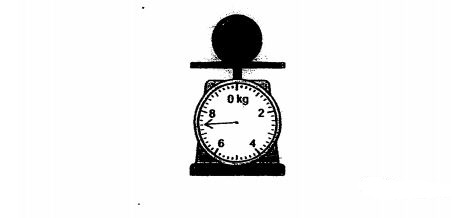# Question 1 of 50

Alfred had $\frac{7}{8}$kg of flour. He packed them into smaller packets of $\frac{1}{4}$kg each. What is the mass of the remaining flour? $\frac{1}{8}$ 11. What is the mass of the ball on the weighting scale?A
6kg 200g
B
6kg 400g
C
7kg 200g
D
7kg 400g
E
None of the above